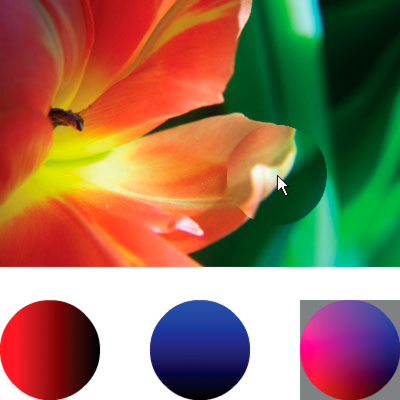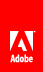# Displacement map filter

Flash Player 9 and later, Adobe AIR 1.0 and later

 The DisplacementMapFilter class uses pixel values from a BitmapData object (known as the displacement map image) to perform a displacement effect on a new object. The displacement map image is typically different than the actual display object or BitmapData instance to which the filter is being applied. A displacement effect involves displacing pixels in the filtered image—in other words, shifting them away from their original location to some extent. This filter can be used to create a shifted, warped, or mottled effect. The location and amount of displacement applied to a given pixel is determined by the color value of the displacement map image. When working with the filter, in addition to specifying the map image, you specify the following values to control how the displacement is calculated from the map image: Map point: The location on the filtered image at which the upper-left corner of the displacement filter will be applied. You can use this if you only want to apply the filter to part of an image. X component: Which color channel of the map image affects the x position of pixels. Y component: Which color channel of the map image affects the y position of pixels. X scale: A multiplier value that specifies how strong the x axis displacement is. Y scale: A multiplier value that specifies how strong the y axis displacement is. Filter mode: Determines what to do in any empty spaces created by pixels being shifted away. The options, defined as constants in the DisplacementMapFilterMode class, are to display the original pixels (filter mode IGNORE ), to wrap the pixels around from the other side of the image (filter mode WRAP , which is the default), to use the nearest shifted pixel (filter mode CLAMP ), or to fill in the spaces with a color (filter mode COLOR ). To understand how the displacement map filter works, consider a basic example. In the following code, an image is loaded, and when it finishes loading it is centered on the Stage and a displacement map filter is applied to it, causing the pixels in the entire image to shift horizontally to the left. ```import flash.display.BitmapData; import flash.display.Loader; import flash.events.MouseEvent; import flash.filters.DisplacementMapFilter; import flash.geom.Point; import flash.net.URLRequest; // Load an image onto the Stage. var loader:Loader = new Loader(); var url:URLRequest = new URLRequest("http://www.helpexamples.com/flash/images/image3.jpg"); loader.load(url); this.addChild(loader); var mapImage:BitmapData; var displacementMap:DisplacementMapFilter; // This function is called when the image finishes loading. function setupStage(event:Event):void { // Center the loaded image on the Stage. loader.x = (stage.stageWidth - loader.width) / 2; loader.y = (stage.stageHeight - loader.height) / 2; // Create the displacement map image. mapImage = new BitmapData(loader.width, loader.height, false, 0xFF0000); // Create the displacement filter. displacementMap = new DisplacementMapFilter(); displacementMap.mapBitmap = mapImage; displacementMap.mapPoint = new Point(0, 0); displacementMap.componentX = BitmapDataChannel.RED; displacementMap.scaleX = 250; loader.filters = [displacementMap]; } loader.contentLoaderInfo.addEventListener(Event.COMPLETE, setupStage);``` The properties used to define the displacement are as follows: Map bitmap: The displacement bitmap is a new BitmapData instance created by the code. Its dimensions match the dimensions of the loaded image (so the displacement is applied to the entire image). It is filled with solid red pixels. Map point: This value is set to the point 0, 0—again, causing the displacement to be applied to the entire image. X component: This value is set to the constant BitmapDataChannel.RED , meaning the red value of the map bitmap will determine how much the pixels are displaced (how much they move) along the x axis. X scale: This value is set to 250. The full amount of displacement (from the map image being completely red) only displaces the image by a small amount (roughly one-half of a pixel), so if this value was set to 1 the image would only shift .5 pixels horizontally. By setting it to 250, the image shifts by approximately 125 pixels. These settings cause the filtered image’s pixels to shift 250 pixels to the left. The direction (left or right) and amount of shift is based on the color value of the pixels in the map image. Conceptually, the filter goes through the pixels of the filtered image one by one (at least, the pixels in the region where the filter is applied, which in this case means all the pixels), and does the following with each pixel: It finds the corresponding pixel in the map image. For example, when the filter calculates the displacement amount for the pixel in the upper-left corner of the filtered image, it looks at the pixel in the upper-left corner of the map image. It determines the value of the specified color channel in the map pixel. In this case, the x component color channel is the red channel, so the filter looks to see what the value of the red channel of the map image is at the pixel in question. Since the map image is solid red, the pixel’s red channel is 0xFF, or 255. This is used as the displacement value. It compares the displacement value to the “middle” value (127, which is halfway between 0 and 255). If the displacement value is lower than the middle value, the pixel shifts in a positive direction (to the right for x displacement; down for y displacement). On the other hand, if the displacement value is higher than the middle value (as in this example), the pixel shifts in a negative direction (to the left for x displacement; up for y displacement). To be more precise, the filter subtracts the displacement value from 127, and the result (positive or negative) is the relative amount of displacement that is applied. Finally, it determines the actual amount of displacement by determining what percentage of full displacement the relative displacement value represents. In this case, full red means 100% displacement. That percentage is then multiplied by the x scale or y scale value to determine the number of pixels of displacement that will be applied. In this example, 100% times a multiplier of 250 determines the amount of displacement—roughly 125 pixels to the left. Because no values are specified for y component and y scale, the defaults (which cause no displacement) are used—that’s why the image doesn’t shift at all in the vertical direction. The default filter mode setting, WRAP , is used in the example, so as the pixels shift to the left the empty space on the right is filled in by the pixels that shifted off the left edge of the image. You can experiment with this value to see the different effects. For example, if you add the following line to the portion of code where the displacement properties are being set (before the line loader.filters = [displacementMap] ), it will make the image look as though it has been smeared across the Stage: `displacementMap.mode = DisplacementMapFilterMode.CLAMP;` For a more complex example, the following listing uses a displacement map filter to create a magnifying glass effect on an image: ```import flash.display.Bitmap; import flash.display.BitmapData; import flash.display.BitmapDataChannel; import flash.display.GradientType; import flash.display.Loader; import flash.display.Shape; import flash.events.MouseEvent; import flash.filters.DisplacementMapFilter; import flash.filters.DisplacementMapFilterMode; import flash.geom.Matrix; import flash.geom.Point; import flash.net.URLRequest; // Create the gradient circles that will together form the // displacement map image var radius:uint = 50; var type:String = GradientType.LINEAR; var redColors:Array = [0xFF0000, 0x000000]; var blueColors:Array = [0x0000FF, 0x000000]; var alphas:Array = [1, 1]; var ratios:Array = [0, 255]; var xMatrix:Matrix = new Matrix(); xMatrix.createGradientBox(radius * 2, radius * 2); var yMatrix:Matrix = new Matrix(); yMatrix.createGradientBox(radius * 2, radius * 2, Math.PI / 2); var xCircle:Shape = new Shape(); xCircle.graphics.lineStyle(0, 0, 0); xCircle.graphics.beginGradientFill(type, redColors, alphas, ratios, xMatrix); xCircle.graphics.drawCircle(radius, radius, radius); var yCircle:Shape = new Shape(); yCircle.graphics.lineStyle(0, 0, 0); yCircle.graphics.beginGradientFill(type, blueColors, alphas, ratios, yMatrix); yCircle.graphics.drawCircle(radius, radius, radius); // Position the circles at the bottom of the screen, for reference. this.addChild(xCircle); xCircle.y = stage.stageHeight - xCircle.height; this.addChild(yCircle); yCircle.y = stage.stageHeight - yCircle.height; yCircle.x = 200; // Load an image onto the Stage. var loader:Loader = new Loader(); var url:URLRequest = new URLRequest("http://www.helpexamples.com/flash/images/image1.jpg"); loader.load(url); this.addChild(loader); // Create the map image by combining the two gradient circles. var map:BitmapData = new BitmapData(xCircle.width, xCircle.height, false, 0x7F7F7F); map.draw(xCircle); var yMap:BitmapData = new BitmapData(yCircle.width, yCircle.height, false, 0x7F7F7F); yMap.draw(yCircle); map.copyChannel(yMap, yMap.rect, new Point(0, 0), BitmapDataChannel.BLUE, BitmapDataChannel.BLUE); yMap.dispose(); // Display the map image on the Stage, for reference. var mapBitmap:Bitmap = new Bitmap(map); this.addChild(mapBitmap); mapBitmap.x = 400; mapBitmap.y = stage.stageHeight - mapBitmap.height; // This function creates the displacement map filter at the mouse location. function magnify():void { // Position the filter. var filterX:Number = (loader.mouseX) - (map.width / 2); var filterY:Number = (loader.mouseY) - (map.height / 2); var pt:Point = new Point(filterX, filterY); var xyFilter:DisplacementMapFilter = new DisplacementMapFilter(); xyFilter.mapBitmap = map; xyFilter.mapPoint = pt; // The red in the map image will control x displacement. xyFilter.componentX = BitmapDataChannel.RED; // The blue in the map image will control y displacement. xyFilter.componentY = BitmapDataChannel.BLUE; xyFilter.scaleX = 35; xyFilter.scaleY = 35; xyFilter.mode = DisplacementMapFilterMode.IGNORE; loader.filters = [xyFilter]; } // This function is called when the mouse moves. If the mouse is // over the loaded image, it applies the filter. function moveMagnifier(event:MouseEvent):void { if (loader.hitTestPoint(loader.mouseX, loader.mouseY)) { magnify(); } } loader.addEventListener(MouseEvent.MOUSE_MOVE, moveMagnifier);``` The code first generates two gradient circles, which are combined together to form the displacement map image. The red circle creates the x axis displacement ( xyFilter.componentX = BitmapDataChannel.RED ), and the blue circle creates the y axis displacement ( xyFilter.componentY = BitmapDataChannel.BLUE ). To help you understand what the displacement map image looks like, the code adds the original circles as well as the combined circle that serves as the map image to the bottom of the screen.The code then loads an image and, as the mouse moves, applies the displacement filter to the portion of the image that’s under the mouse. The gradient circles used as the displacement map image causes the displaced region to spread out away from the pointer. Notice that the gray regions of the displacement map image don’t cause any displacement. The gray color is 0x7F7F7F . The blue and red channels of that shade of gray exactly match the middle shade of those color channels, so there is no displacement in a gray area of the map image. Likewise, in the center of the circle there is no displacement. Although the color there isn’t gray, that color’s blue channel and red channel are identical to the blue channel and red channel of medium gray, and since blue and red are the colors that cause displacement, no displacement happens there.Twitter™ and Facebook posts are not covered under the terms of Creative Commons.// Ethnio survey code removed